# Posts published in “Medium”

Given a char array representing tasks CPU need to do. It contains capital letters A to Z where different letters represent different tasks.Tasks could be done without original order. Each task could be done in one interval. For each interval, CPU could finish one task or just be idle.

However, there is a non-negative cooling interval n that means between two same tasks, there must be at least n intervals that CPU are doing different tasks or just be idle.

You need to return the least number of intervals the CPU will take to finish all the given tasks.

Example 1:

Note:

1. The number of tasks is in the range [1, 10000].
2. The integer n is in the range [0, 100].

Idea:

Counting

Time complexity: O(n)

Space complexity: O(1)

Problem:

Given a non-empty array of integers, return the k most frequent elements.

For example,
Given [1,1,1,2,2,3] and k = 2, return [1,2].

Note:
You may assume k is always valid, 1 ≤ k ≤ number of unique elements.
Your algorithm’s time complexity must be better than O(n log n), where n is the array’s size.

Idea: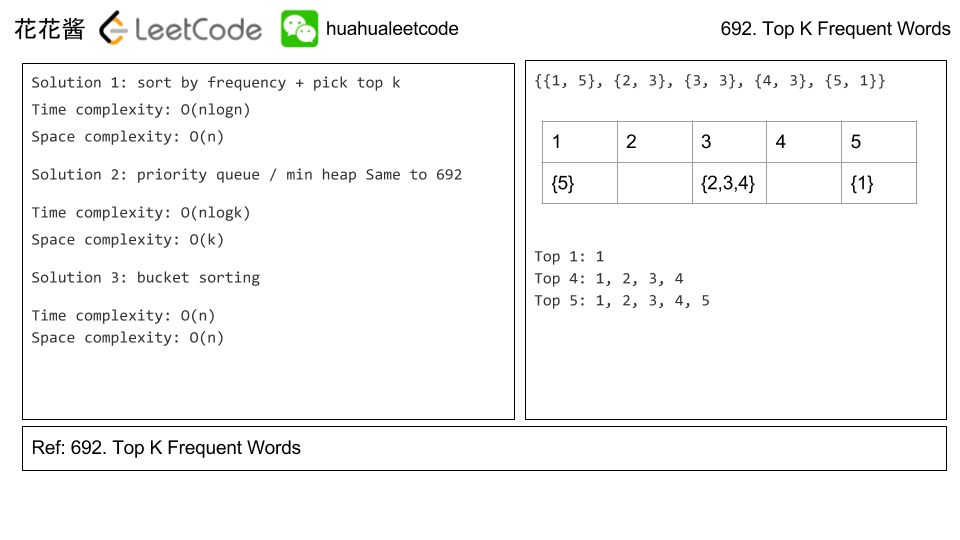# Solution 2: Priority queue / max heap

Time complexity: O(n) + O(nlogk)

Space complexity: O(n)

# Solution 3: Bucket Sort

Time complexity: O(n)

Space complexity: O(n)

# Related Problems

Problem:

Given a non-empty list of words, return the k most frequent elements.

Your answer should be sorted by frequency from highest to lowest. If two words have the same frequency, then the word with the lower alphabetical order comes first.

Example 1:

Example 2:

Note:

1. You may assume k is always valid, 1 ≤ k ≤ number of unique elements.
2. Input words contain only lowercase letters.

1. Try to solve it in O(n log k) time and O(n) extra space.

Idea:

Priority queue / min heap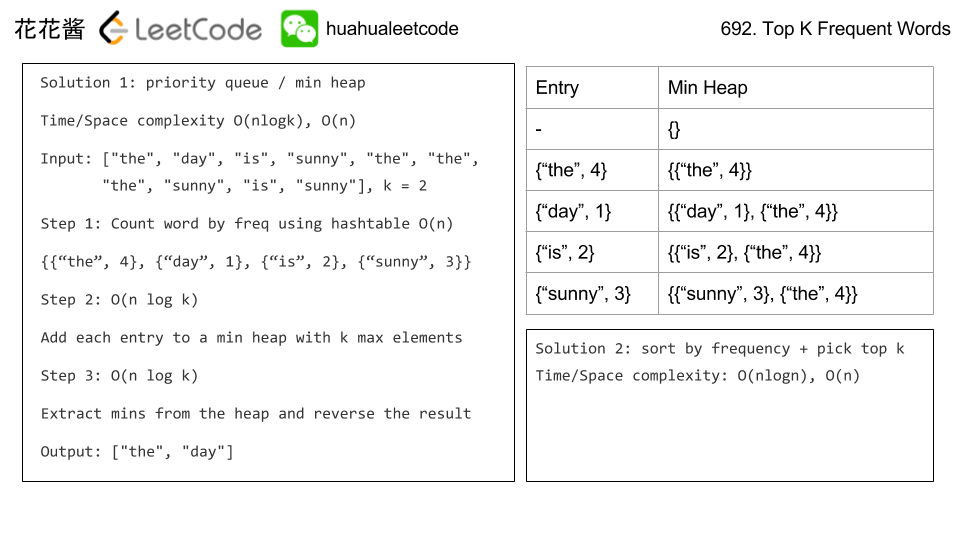Solution

C++ / priority_queue O(n log k) / O(n)

Related Problems

Problem:

There are a total of n courses you have to take, labeled from 0 to n - 1.

Some courses may have prerequisites, for example to take course 0 you have to first take course 1, which is expressed as a pair: [0,1]

Given the total number of courses and a list of prerequisite pairs, is it possible for you to finish all courses?

For example:

There are a total of 2 courses to take. To take course 1 you should have finished course 0. So it is possible.

There are a total of 2 courses to take. To take course 1 you should have finished course 0, and to take course 0 you should also have finished course 1. So it is impossible.

Idea:

Finding cycles O(n^2) -> Topological sort O(n)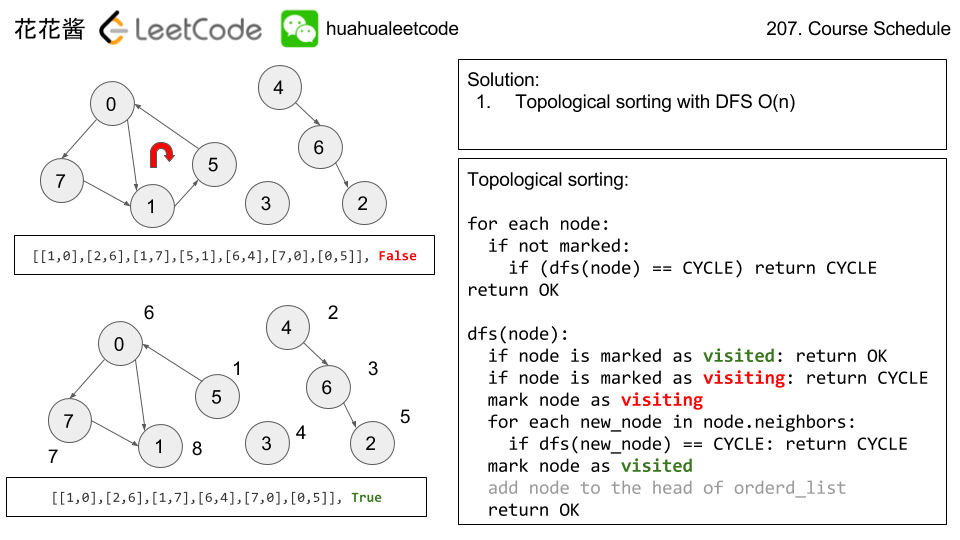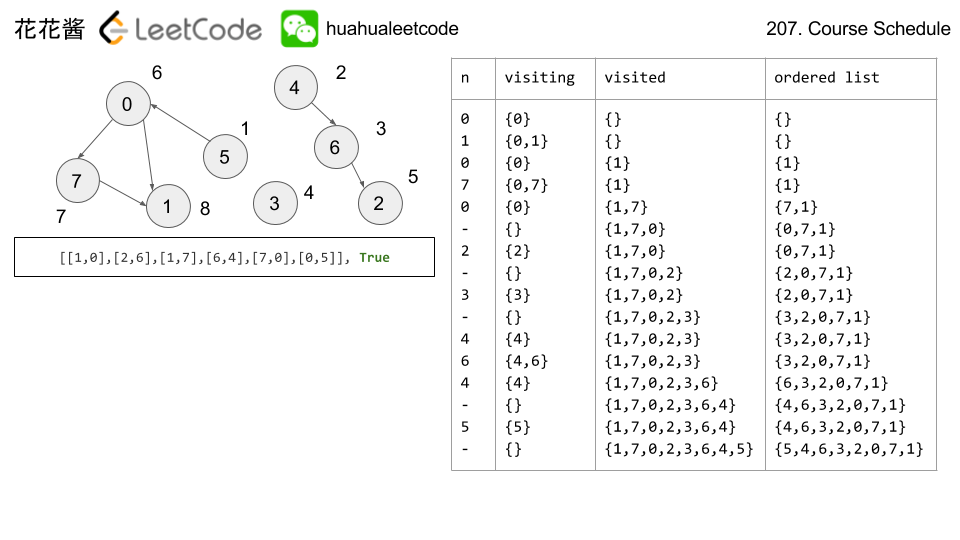# Solution 2: DFS Finding cycles

Time complexity: O(n^2)

Space complexity: O(n)

# Related Pr0blems:

Problem:

Serialization is the process of converting a data structure or object into a sequence of bits so that it can be stored in a file or memory buffer, or transmitted across a network connection link to be reconstructed later in the same or another computer environment.

Design an algorithm to serialize and deserialize a binary search tree. There is no restriction on how your serialization/deserialization algorithm should work. You just need to ensure that a binary search tree can be serialized to a string and this string can be deserialized to the original tree structure.

The encoded string should be as compact as possible.

Note: Do not use class member/global/static variables to store states. Your serialize and deserialize algorithms should be stateless.

Idea: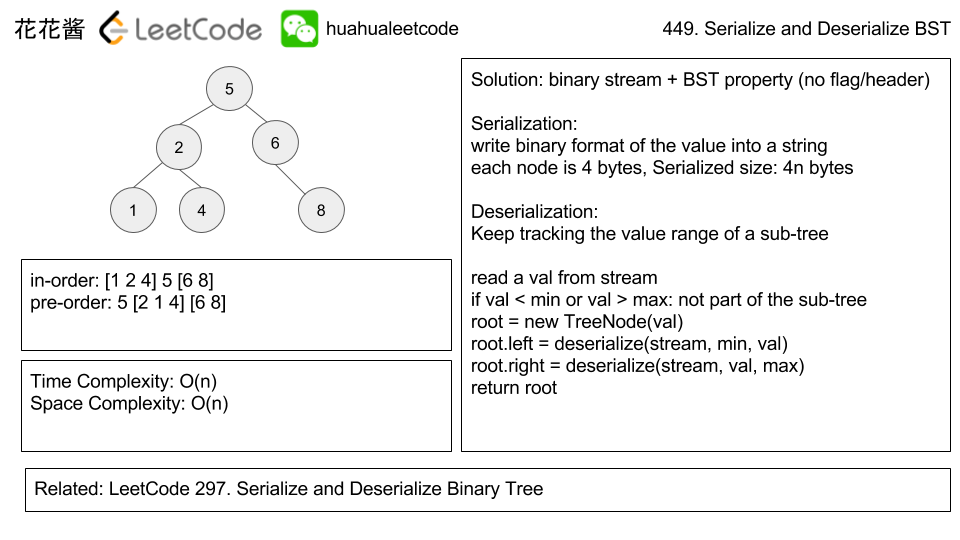Binary format

serialized size: 4*n bytes, n is the number of nodes in the BST.

Time complexity: O(n)

Space complexity: O(n)

Solution:

C++

Related Problems

Mission News Theme by Compete Themes.